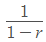## Monday, May 13, 2019

### Advanced Math Solutions - Series Convergence Calculator, Geometric Series

Series are an important part of Calculus. In this next series of blog posts, I will be discussing infinite series and how to determine if they converge or diverge.

For a refresher:

A series is the sum of a list of terms that are generated with a pattern. A series is denoted with a summation symbol. An infinite series is a series that has an infinite number of terms being added together.

Here is an example of an infinite series:

With infinite series, it can be hard to determine if the series converges or diverges. Luckily, there are convergence tests to help us determine this!

In this blog post, I will go over the convergence test for geometric series, a type of infinite series.

A geometric series is a series that has a constant ratio between successive terms. A visualization of this will help you better understand.

Here’s a geometric series:

In this series, each following term is the product of the prior term and ⅓.

We can rewrite this geometric series using the summation notation.

In order to determine if a geometric series diverges or converges, you’ll need to follow and remember the following test/rule:

If the series is of the form,

if |r|<1, then the geometric series converges toif |r|≥1, then the geometric series diverges

Let’s see some examples to better understand.

1. Reference the geometric series convergence test

2. Determine the value of r

3. Determine if the series converges or diverges

The geometric series converges to \frac{5}{4}.

1. Reference the geometric series convergence test

2. Determine the value of r

3. Determine if the series converges or diverges

The geometric series diverges.

1. Reference the geometric series convergence test

2. Determine the value of r

3. Determine if the series converges or diverges

The geometric series converges to 6.

As you can see, it is not too difficult to determine if a geometric series converges or not. After doing some practice problems, you’ll get the hang of it very quickly. For more help or practice on geometric series, check out Symbolab’s Practice. Next blog post, I’ll go over the convergence test for p-series.

Until next time,

Leah